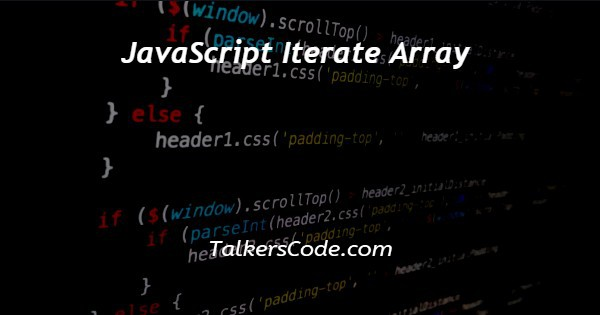# JavaScript Iterate Array

Last Updated : Jan 1, 2023In this tutorial we will show you the solution of JavaScript iterate array, here, iteration means to use loops on array inside JavaScript. One main thing to note here that there are many ways with help of which we are able to iterate array in JavaScript.

We are able to show only a few from them in this article, and remaining are discussed in our next tutorials. Because it is not possible to understand all of them in one article.

## Step By Step Guide On JavaScript Iterate Array :-

As, we already said that there are many ways to iterate an array in JavaScript.

And we will understand a few in each tutorial, remaining are discussed in our next tutorial. So, now let us start our iterating session and understand from basic way to use iterations on array to advance.

Here, in the example given below we are going to use while, for and for each loop to array. Let us see how to use for loop in code.

```// iterate array in JavaScript using while loop
<script>
i = 0;
array = [ 1, 2, 3, 4, 5 ];
while (i < array.length) {
console.log(array[i]);
i++;
}
</script>
// iterate array in JavaScript using for loop
<script>
array = [ 1, 2, 3, 4, 5];
for (i = 0; i < array.length; i++) {
console.log(array[i]);
}
</script>
// iterate array in JavaScript using for each loop
<script>
i = 0;
array = [ 1, 2, 3, 4, 5];
array.forEach(result);
function result(item, i)
{
console.log(item);
}
</script>```
1. Here, above we show you an example in JavaScript.
2. We can also used basic html codes here to use these iteration inside html codes. We hope you all know that what is the basic html structure and how to write this JavaScript code inside html tags.
3. If you don’t know anything from above you can visit to our article, in which you are able to learn these concepts easily from our experienced developers.
4. Now, let us talk about our codes. As, above we already said that we for iterations we use only while, for and for each loop in this article. And we think that what is the meaning of these loop means which loop is used for which purpose. This basic knowledge of loop helps you easily understand above example.
5. Now, as we see above that in first two we create an array and then use array.length to get number of elements of array. These all helps us iterate array using for and while loop. Whereas in case of for each we just use its key to iterate arrays.

## Conclusion :-

At last in conclusion, here we can say that with the help of this article we are able to understand how to get cookies by name using JavaScript.

I hope this tutorial on JavaScript iterate array helps you and the steps and method mentioned above are easy to follow and implement.